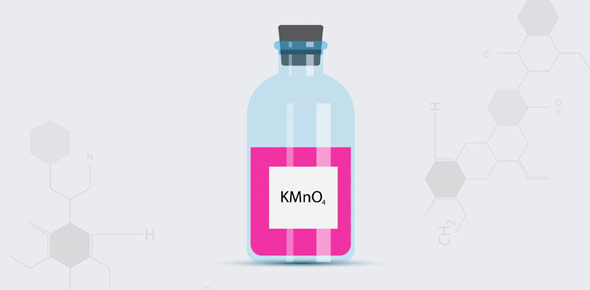# Unit 9 - Strength Of Acids And Bases

6 Questions | Total Attempts: 464SettingsIn chemistry, acids and bases are two types of corrosive substances. The acidity or basicity of a substance is measured with the pH scale. What can you tell us about the strengths of these two corrosives?

• 1.
The strength of an acid or base depends on ______.
• A.

How many hydrogen atoms are contained in each molecule of the acid or base.

• B.

How quickly they form salts when they react with metals

• C.

How completely it ionizes in water

• D.

How many hydroxide groups are on each molecule of the acid or base

• 2.
Which two terms refer to the amount of acid or base dissolved in solution?
• A.

Dilute and concentrated

• B.

Strong and dilute

• C.

Weak and concentrated

• D.

Strong and weak

• 3.
The ______ of solution is a measure of the concentration of hydronium ions in it.
• A.

Concentration

• B.

Indicator

• C.

PH

• D.

Strength

• 4.
Which of the following has the highest pH?
• A.

Pure water

• B.

Vinegar

• C.

Ammonia

• D.

Vitamin C

• 5.
______ are solution containing ions that react with acids or bases to minimize their effects.
• A.

Buffers

• B.

Enzymes

• C.

Indicators

• D.

Hydroxides

• 6.
In chemical equations describing ionization, what do double arrows pointing in opposite directions indicate?
• A.

The reaction rate is doubled.

• B.

There is twice as much product as reactant.

• C.

The reactant is a strong acid.

• D.

The ionization reaction is incomplete.

Related TopicsBack to top